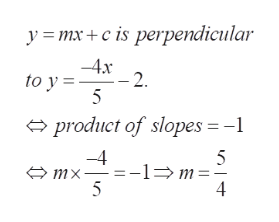# Find the equation of the line that contains the pointP(−4, −3)and is perpendicular to the graph ofy = − 4/5x − 2.

Question
6 views

Find the equation of the line that contains the point

P(−4, −3)

and is perpendicular to the graph of

y = − 4/5x − 2.

check_circle

Step 1

To determine the equation of the straight line satisfying the given properties

Step 2

The equation of a straight line is of the form y=mx+c, where m represents the slope of the line. Determine m from the perpendicularity conditionhelp_outlineImage Transcriptioncloseymx+cis perpendicular 4x - 2 to y 5 product of slopes = -1 -4 -1m 5 5 mx 4 fullscreen
Step 3

To find c, use the fact that the line passes through P(-4,-3)...

### Want to see the full answer?

See Solution

#### Want to see this answer and more?

Solutions are written by subject experts who are available 24/7. Questions are typically answered within 1 hour.*

See Solution
*Response times may vary by subject and question.
Tagged in

### Algebra